### Nonlinear Systems of Equations: Problems

1. Use your implementation of the Newton-Raphson method to find 4 different sets of roots for the following nonlinear system of equations: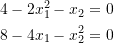Use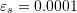. Compare with the “FindRoot” built-in function in Mathematica.
2. Use your implementation of the Newton-Raphson method to find 1 set of roots for the following nonlinear system of equations: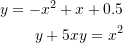Use. Compare with the “FindRoot” built-in function in Mathematica.
3. Compare the convergence of the Newton-Raphson method and the fixed-point iteration method with initial guesses of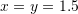andto find a solution to the following nonlinear system of equations: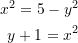4. Use the Newton-Raphson method to find a solution to the following nonlinear set of equations: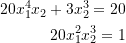Use5. The following structural system is composed of two truss elements with unit cross sectional area. The left and right supports are hinged supports. The middle connection where the load is applied is also a hinged connection. Find the displacement at equilibrium of the following structural system. Assume a linear material with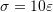whereis the stress in the bar,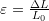is the corresponding strain, and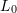is the initial length. Use the Newton-Raphson method.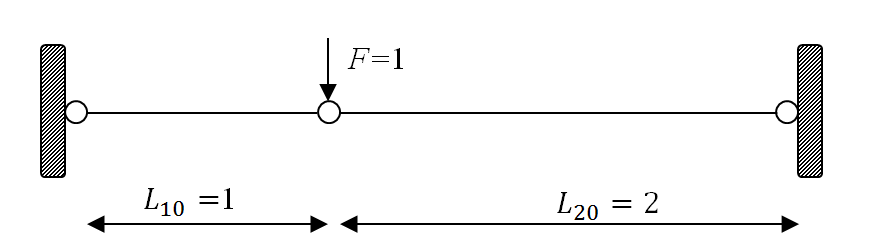6. Solve the following nonlinear system of equations using the Newton-Raphson method and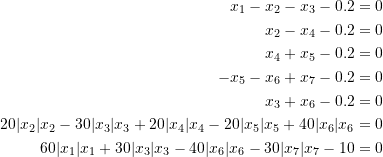Hint, if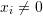: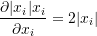7. The following three equations describe a plane, a hyperbolic paraboloid (i.e., a saddle function), and a sphere, respectively.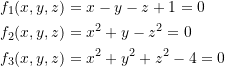Find the coordinates of the two points at which the three surface plots intersect.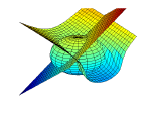3D plot of the sphere, the paraboloid, and the plane intersecting each other

8. Solve the following system of nonlinear equations using the Newton-Raphson method.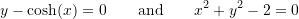Plot the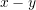curves for each equation.

9. Use the Newton-Raphson method to solve the following linear system of equations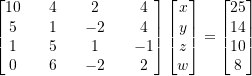Provide comments on the behaviour of this method for a linear system of equations with regards to the solution procedure and number of iterations to reach a certain accuracy. Explain the reasons or causes of this behaviour.